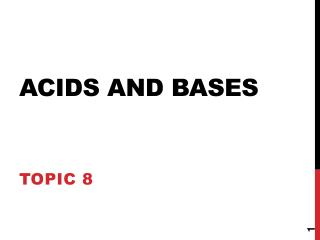DownloadDownload PresentationAcids and Bases

Acids and Bases

Télécharger la présentationAcids and Bases

- - - - - - - - - - - - - - - - - - - - - - - - - - - E N D - - - - - - - - - - - - - - - - - - - - - - - - - - -
Presentation Transcript

1. Acids and Bases Topic 8

2. Arrhenius Model of Acids and Bases • Acid is a substance that contains hydrogen and ionizes to produce a hydrogen ion in an aqueous solution. • Base is a substance that contains a hydroxide group (OH) and dissociates to produce a hydroxide ion in aqueous solution. • This model did not include all bases (some bases (ammonia) do not have a OH group)

3. 8.1.1-8.1.2Lewis Model of Acids & Bases • A Lewis acid is a substance that can accept a pair of electrons to form a covalent bond. • A Lewis base is a substance that can donate an electron pair to form a covalent bond.

4. 8.1.1-8.1.2 Brønsted-Lowry Model of Acids and Bases • Acid is a substance that donates an H+ ion (proton) to another substance. • Base is a substance that accepts an H+ ion (proton) from another substance.

5. 8.1.3 Conjugate Acids and Bases • After an acid donates an H+, it becomes a conjugate base. • After a base accepts an H+, it becomes a conjugate acid. HCl + H2O  H3O+ + Cl-

6. 8.2 Properties of Acids and BAses • Acids and bases can be identified using indicators. • Indicators change color according to concentration of H+ ions.

7. Acids react to form salts • A salt is a compound formed when the H of an acid is replaced by a metal or other positive ion. • There are 3 main types: • Acid + metal  salt + hydrogen • 2HCl + Zn  ZnCl2 + H2 • Acid + base  salt + water • HCl + NaOH  NaCl + H2O • Acid + carbonate  salt + water + carbon dioxide • 2HCl + CaCO3  CaCl2 + H2O + CO2

8. 8.3 Strong and Weak Acids/Bases • Generally divide acids and bases into STRONG or WEAK ones. STRONG ACID: HNO3(aq) + H2O(liq)  H3O+(aq) + NO3-(aq) HNO3 is about 100% dissociated in water.

9. Strong and Weak Acids/Bases HNO3, HCl, H2SO4 and HClO4 are among the only known strong acids.

10. Strong and Weak Acids/Bases • Weak acids are much less than 100% ionized in water. One of the best known is acetic acid = CH3CO2H

11. CaO Strong and Weak Acids/Bases • Strong Base: 100% dissociated in water. NaOH(aq)  Na+(aq) + OH-(aq) Other common strong bases include KOH and Ca(OH)2. CaO (lime) + H2O  Ca(OH)2 (slaked lime)

12. Strong and Weak Acids/Bases • Weak base: less than 100% ionized in water One of the best known weak bases is ammonia NH3(aq) + H2O(liq)  NH4+(aq) + OH-(aq)

13. 8.4 the pH Scale A common way to express acidity and basicity is with pH pH =- log [H+] In a neutral solution, [H3O+] = [OH-] = 1.00 x 10-7 at 25 oC pH = -log (1.00 x 10-7) = - (-7) = 7

14. If the pH of Diet Coke is 3.12, what is it’s H+concentration? Because pH = - log [H+] then log [H+] = - pH Take antilog and get [H+] = 10-pH [H+] = 10-3.12 = 7.6 x 10-4 M

15. Convenient features of ph scale • pH numbers are usually positive and have no units • The pH number is inversely related to the [H+] • A change in one pH unit represents a 10 fold change in [H+]